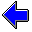Java Security Settings:
This web page employs Java, which requires specific security settings for correct operation.
If the applets on this page do not run correctly, consult the Virtual Chemistry Experiments FAQ
or the Physlet Physics web site for establishing the correct security settings.

# Gas Laws

## Dalton's Law of Partial Pressures

### Concepts

One of the important predictions made by Avogadro is that the identity of a gas is unimportant in determining the P-V-T properties of the gas. This behavior means that a gas mixture behaves in exactly the same fashion as a pure gas. (Indeed, early scientists such as Robert Boyle studying the properties of gases performed their experiments using gas mixtures, most notably air, rather than pure gases.)

The ideal gas law,

P V = n R T ,

predicts how the pressure, volume, and temperature of a gas depend upon the number of moles of the gas. The number of moles, n, is the total moles of all the gas-phase species.

Air, for example, is composed primarily of nitrogen and oxygen. In a given sample of air, the total number of moles is can be approximated as

n = nnitrogen + noxygen

This expression for n can be substituted into the ideal gas law to yield

P V = ( nnitrogen + noxygen ) R T

All molecules in the gas have access to the entire volume of the system, thus V is the same for both nitrogen and oxygen. Similarly, both compounds experience the same temperature. One can therefore split this expression of the ideal gas law into two terms, one for nitrogen and one for oxygen.

P = nnitrogen R T/V + noxygen R T/V

P = Pnitrogen + Poxygen

The above equation is called Dalton's Law of Partial Pressure, and it states that the pressure of a gas mixture is the sum of the partial pressures of the individual components of the gas mixture. Pnitrogen is the partial pressure of the nitrogen and Poxygen is the partial pressure of oxygen.

Pnitrogen = nnitrogen R T/V

Poxygen = noxygen R T/V

You'll notice that the equations for the partial pressures are really just the ideal gas law, but the moles of the individual component (nitrogen or oxygen) are used instead of the total moles. Conceptually Pnitrogen is the contribution nitrogen molecules make to the pressure and Poxygen is the contribution oxygen molecules make.

### Exercise

Objective

• Employ Boyle's Law and Dalton's Law of Partial Pressures to predict the pressure of a gas mixture.

This exercise involves the same two-bulb apparatus employed in a previous exercise. The left bulb is filled with nitrogen gas (artificially colored blue) and the right bulb is filled with oxygen gas (artificially colored red). The volumes of the two bulbs are indicated and manometers are provided to measure the pressure inside each bulb. Each time you press the "New Conditions" button, the experiment will be reset with new volumes and initial pressures for the two bulbs.

When the stopcock is open, the nitrogen will expand into the right bulb and the oxygen into the left bulb to produce a uniform mixture.

Use Boyle's Law to calculate the partial pressure of nitrogen and the partial pressure of oxygen in the final gas mixture. Use these partial pressures and Dalton's Law to calculate the pressure for the gas mixture.

Open the stopcock and measure the final pressure. Was your calculation accurate?

Practice the calculations until you are able to solve the problem consistently.

 Volume of Left Bulb L Volume of Right Bulb LIdeal Gas Law and the Gas ConstantGas Laws Home PageVirtual Chemistry Home Page

DaltonsLaw.html version 2.1